# Test: Frequency Domain Analysis of Control Systems

## 10 Questions MCQ Test Topicwise Question Bank for Electronics Engineering | Test: Frequency Domain Analysis of Control Systems

Description
Attempt Test: Frequency Domain Analysis of Control Systems | 10 questions in 30 minutes | Mock test for Electrical Engineering (EE) preparation | Free important questions MCQ to study Topicwise Question Bank for Electronics Engineering for Electrical Engineering (EE) Exam | Download free PDF with solutions
QUESTION: 1

Solution:
QUESTION: 2

Solution:
QUESTION: 3

### The initial slope of Bode plot for a transfer function having no poles at origin is

Solution:

With no-pole at origin, initial slope will be OdB/decade.
For n-pole at origin, initial slope will be -20 ndB/decade.

QUESTION: 4

The gain margin of a control system having the loop transfer function G(s)H(s) =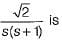Solution: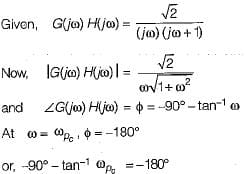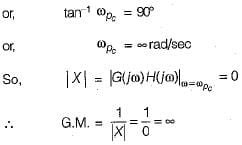QUESTION: 5

Gain crossover frequency is the frequency at which the gain of G(jω) is

Solution: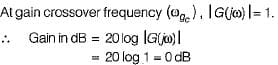QUESTION: 6

For a control system having gain margin of -10 dB, the magnitude of GH(s) for 180° phase shift is

Solution: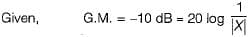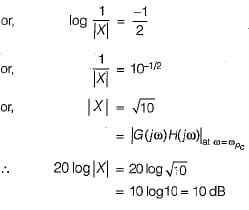QUESTION: 7

If the Nyquist plot of the loop transfer function G(s)H(s) of a closed-loop system does not encloses the (-1, j0) point in the G(s)H(s) plane, the gain margin of the system will be

Solution:

Since the critical point (-1 + j0 ) is not enclosed, therefore m agnitude of OLTF,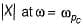will be less than unity.
Therefore, G.M. = 20 log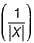will be positive and the system will be stable.

QUESTION: 8

A system with phase margin close to zero or gain margin close to unity is

Solution:
QUESTION: 9

If the transfer function of a first-order system in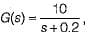then the time constant of this system will be

Solution: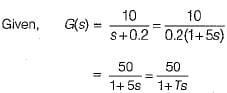Hence, time constant = T= 5 seconds

QUESTION: 10

The polar plot of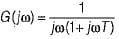Solution: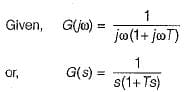Given system is of type-1 and order-2, therefore its polar plot will be as shown below.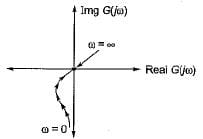Hence, the polar plot will neither cross the real axis nor the imaginary axis.Use Code STAYHOME200 and get INR 200 additional OFF Use Coupon Code